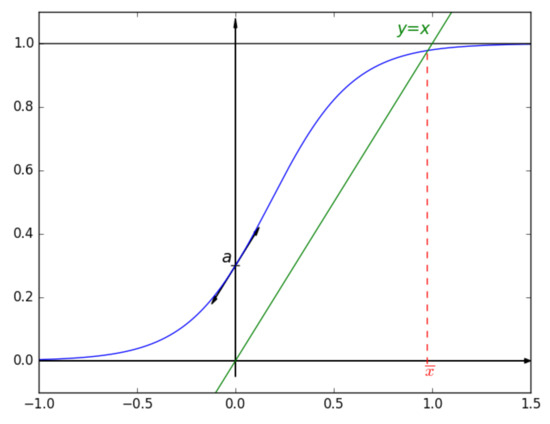Next Article in Journal
Critical Domain Problem for the Reaction–Telegraph Equation Model of Population Dynamics
Next Article in Special Issue
Gray Codes Generation Algorithm and Theoretical Evaluation of Random Walks in N-Cubes
Previous Article in Journal
Quasirecognition by Prime Graph of the Groups 2D2n(q) Where q < 105
Previous Article in Special Issue
Asynchronous Iterations of Parareal Algorithm for Option Pricing Models
Open AccessArticle

Theoretical Study of the One Self-Regulating Gene in the Modified Wagner Model

1
Femto-ST Institute, UMR 6174 CNRS, University of Bourgogne Franche-Comté, 90000 Belfort, France
2
EGCE, CNRS-IRD-Université Paris-Saclay, 91198 Gif-sur-Yvette, France
*
Author to whom correspondence should be addressed.
Mathematics 2018, 6(4), 58; https://doi.org/10.3390/math6040058
Received: 31 January 2018 / Revised: 6 March 2018 / Accepted: 31 March 2018 / Published: 9 April 2018
Predicting how a genetic change affects a given character is a major challenge in biology, and being able to tackle this problem relies on our ability to develop realistic models of gene networks. However, such models are rarely tractable mathematically. In this paper, we propose a mathematical analysis of the sigmoid variant of the Wagner gene-network model. By considering the simplest case, that is, one unique self-regulating gene, we show that numerical simulations are not the only tool available to study such models: theoretical studies can be done too, by mathematical analysis of discrete dynamical systems. It is first shown that the particular sigmoid function can be theoretically investigated. Secondly, we provide an illustration of how to apply such investigations in the case of the dynamical system representing the one self-regulating gene. In this context, we focused on the composite function $f a ( m . x )$ where $f a$ is the parametric sigmoid function and m is a scalar not in ${ 0 , 1 }$ and we have proven that the number of fixed-point can be deduced theoretically, according to the values of a and m. View Full-Text
Show FiguresFigure 1

MDPI and ACS Style

Guyeux, C.; Couchot, J.-F.; Le Rouzic, A.; Bahi, J.M.; Marangio, L. Theoretical Study of the One Self-Regulating Gene in the Modified Wagner Model. Mathematics 2018, 6, 58.

Show more citation formats Show less citations formats
Note that from the first issue of 2016, MDPI journals use article numbers instead of page numbers. See further details here.

1